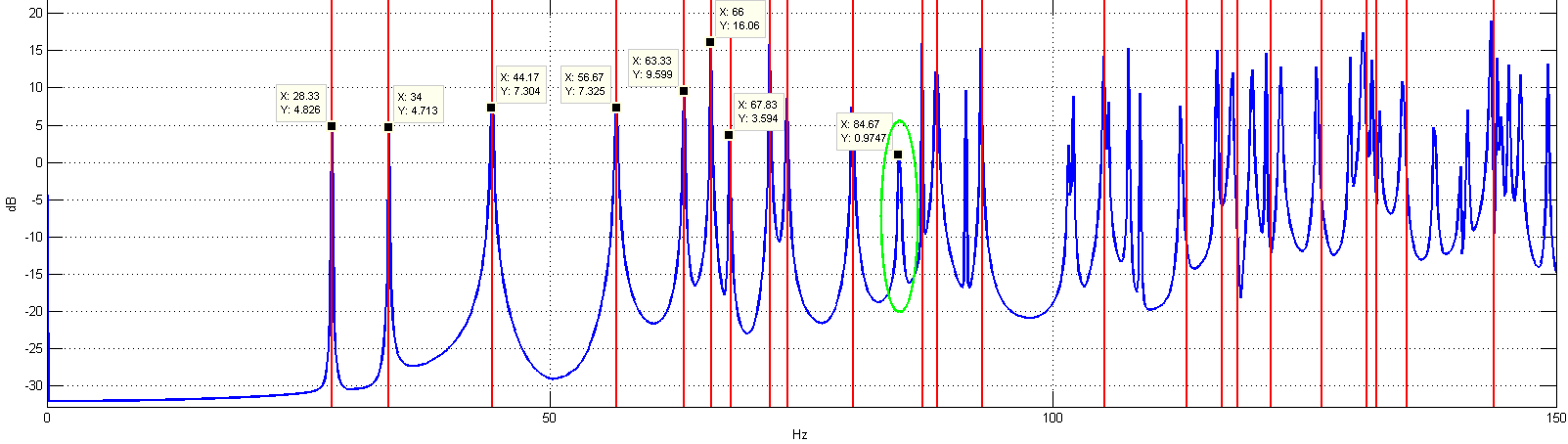# Webpage of Róbert Galambos

Last updated on the 12th of February 2012.

## Introduction

This is my webpage. It is still not ready, but a couple of useful information are already provided. The main goal of this page is to show and make downloadable parts of my work that are freely usable for other. In the future this webpage will evolve as well.

## FDTD simulator for MATLAB

There are a couple of commercial product for FDTD simulations, but I have not found any usable free version of them. Especial not as MATLAB implementation. Therefore I created a some scripts that are simulating acoustic space with the FDTD method. There will be an API documentation in the future, till that you must read the code. It is well commented and formatted.

### Version 0.2.1 beta

Release date: 2012.02.12.
What's new:

• Example in the main.m modified for real parametered rooms.
Featurelist:

• 3D simulation is performed.
• Environment structure can be given as 3D array.
• Possibility of ideal wall simulation.
• Possibility of real wall simulation.
• Wall impedance can varies in the simulation space and is given as the value of the 3D room parameter matrix cell.
Planned for future:
• Open space simulation.
• Absorbing material simulation (like in a silent room).
• Code optimization.

### Version 0.2 beta

Release date: 2012.01.16.
Featurelist:

## API reference

d = RS_GetData( pos ): Get the pressure in the simulation space at the given points.

• pos - A matrix containing on or more return points The form of the matrix is the following for two data points: [ x1, y1, z1; ... x2, y2, z2, ]
• d - Gives back the data at the given points

RS_Init( room , c , p , h , k ): This function initializes the inner structures for the simulations. It must be called before the simulation is used.

• room - The 3D matrix that contains the room and wall parameters.
• 0 - The discrete bin of space is air.
• Z - Any other value represents wall with an impedance of Z.
The grid is h and based on the pressure grid system.
• c - The speed of sound in the simulation.
• p - The constant air pressure.
• h - The grid size (equidistant grid).
• k - The time between an iteration round.

RS_Iterate(): Calculates an iteration of the room with Z wall impedance (real walls).

RS_IterateSimple(): Calculates an iteration of the room with ideal walls.

h = RS_Plot( n , graph , axis , slice , cont , colm ): Draws a slice from the 3D room according to the input parameters.

• n - figure number.
• graph - graph type (velo or pressure) only pressure is available.
• axis - The axis that will be fixed. Can be 'x', 'y', 'z'.
• slice - The slice coordinates of the room in the axis direction.
• cont - The count of the contures.
• colm - The color map of the plot.
• h - The handler to the figure.

RS_SetData( pos ): Set the pressure in the simulation space at the given points.

• pos - A matrix containing on or more excitation points. The form of the marix is the following for two excitation points: [ x1, y1, z1, data1; ... x2, y2, z2, data2 ]

## Validation of simulation

The simulation was run on a 6m x 5m x 3m closed room, with ideal walls to validate the modes of the room. For the validation "Version 0.2.1 beta" was used. You can find the test code in the main.m file.
The simulation run for ~750 seconds and produced the following output (just added some grid after the plot):You can see the theoretical calculated modes in red and the simulation result in blue. The missed peaks (like the green marked) are only becasuse I have marked only the x=0..2, y=0..2, z=0..2 indexed modes.